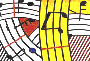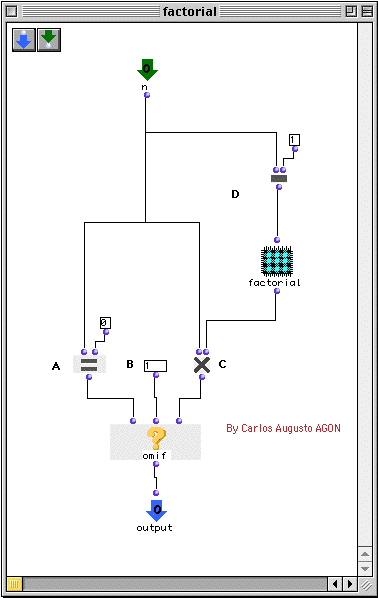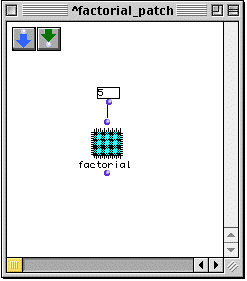Tutorial 38 - Recursive patch I

Topics

Recursive programing with patches

Functions used

omif, om=, om*, om- and omloop

Description

Some computational algorithms require recursive functions. A recursive function is a function in which body we may find a call to itself. A good example is the factorial of an integer. Factorial 5, for example means 1 * 2 * 3 * 4 * 5 and it equals 120. The algorithm maybe something like that:

• First of all we will reverse our list and will multiply 5 * 4 * 3 * 2 * 1
• This will mean that we will start by 5 and multiply it by (5 - 1).
• Then again multiply ((5 - 1) - 1) and so forth i.e ((((5 - 1) - 1) - 1) - 1)
• The recursion will stop until we reach 1. This is the termination test of our recursion. It is important to note thatwithout a termination test inside our function the computation will loop for ever and we will get an error message in the listener such as this:
```> Error: Stack overflow on control stack.
>        To globally increase stack space,
>        increase *minimum-stack-overflow-size*
> While executing: "Unknown"
> Type Command-/ to continue, Command-. to abort.
> If continued: Continue with a larger stack
See the Restarts menu item for further choices.
```
``

Patch structure

First let us create on the workspace an empty patch and add to it one input and one output. The input <n> is our main argument.

A: If n is equal to zero (the termination test) omif (B) will return 1 else, we will multiply n using om* (C) with (n- 1)

D: In order to multiply n with (n- 1), (n- 2), etc... let us drag the patch from the workspace within itself. In this way n will always be tested by the factorial function we created and will be decremented until it equals zero. With every passage through the testing function omif n will be multiplied by (n- 1) and so forth.

Now in order to use this patch drag it onto another patch.The outputs and inputs will appear and we may use it as any other patch. The example above returns 120 which is equal to (5 * 4 * 3 * 2 *1)

NOTE: Another way to do this patch is given in tutorial 36